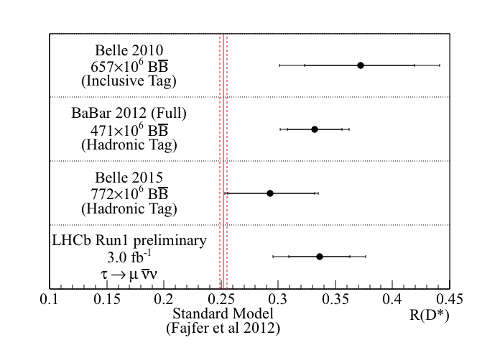### Hints of physics behind standard model?

The LHCb collaboration is studying the decay of mesons $B$ in order to find some violations in standard model rules. In particular LHCb has measured a particular ratio, named $R (D^*)$, between two decay modes of $\overline{B}^0$ and they find a violation from the standard model prediction that is compatible with other similar measures:In the SM all charged leptons, such as taus ($\tau$) or muons ($\mu$), interact in an identical fashion (or, in physicists' language, have the same "couplings"). This property is called "lepton universality". However, differences in mass between the leptons must be accounted for, and affect decays involving these particles. The $\tau$ lepton is much heavier than the $\mu$ lepton and therefore the SM prediction for the ratio $R(D^*)$ is substantially smaller than 1. This ratio is considered to be precisely calculable thanks to the cancellation of uncertainties associated with the $B$ to $D^*$ meson transition.
But there is another hint of new physics. At the end of July Nature Physics published a new paper from the LHCb collaboration about the possible existence of a new particle:
The LHCb collaboration published in Nature Physics a paper based on run 1 data which reports the determination of the parameter $|V_{ub}|$ describing the transition of a $b$ quark to a $u$ quark. This measurement was made by studying a particular decay of the $\Lambda_b^0$ baryon. Other measurements of $|V_{ub}|$ by previous experiments had returned two sets of inconsistent results, depending on which method was used to determine the parameter. Theorists had suggested that this discrepancy could be explained by the presence a new particle contributing to the decay process, which affected the result differently, depending on the measurement method. Today's result from LHCb removes the need for this new particle, while the puzzle of why the original sets of measurements do not agree persists.
where $|V_{ub}|$ is connected to the Cabibbo-Kobayashi-Maskawa matrix.